# NHÀ khoa học nguyễn văN ĐẠo với lý thuyết dao đỘng và chuyểN ĐỘng hỗN ĐỘN

tải về 3.08 Mb.
 trang 7/11 Chuyển đổi dữ liệu 15.11.2017 Kích 3.08 Mb.

## Bifurcation is a concept used to indicate a qualitative change in the features of a dynamical system, such as the number and type of solutions, under the variation of one or more parameters on which the considered system depends. These parameters are called the control parameters, and parameter values at which bifurcations occur are called bifurcation values. A bifurcation diagram is a graph of the state variables versus the parameters [3,6,9].

The bifurcation diagram provides a summary of the essential dynamics and is therefore an important tool for examining the prechaotic or postchaotic changes in a dynamical system under parameter variations. The Poincare’ map can be used to construct the bifurcation diagrams for continuous differential equations. When the data are sampled using a Poincare’ map, it is very easy to observe period doubling and Hopf bifurcations. It is useful because one characteristic precursor to chaotic motion is the appearance of subharmonic periodic vibrations.

We’ll examine two following concrete cases: a) The frequency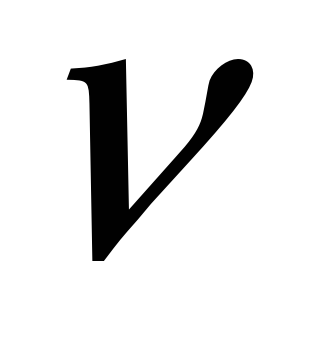is the control parameter b) The forcing amplitude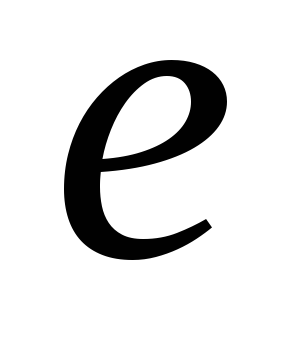is the control parameter.

2.2 The frequencyis the control parameter.

We go back to the system (1) with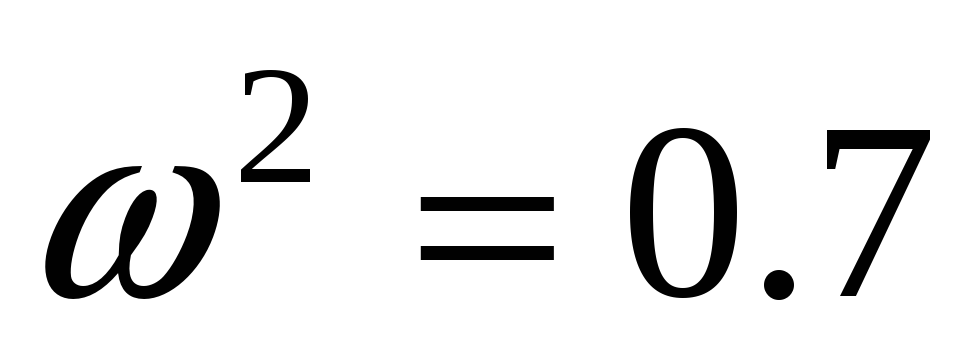,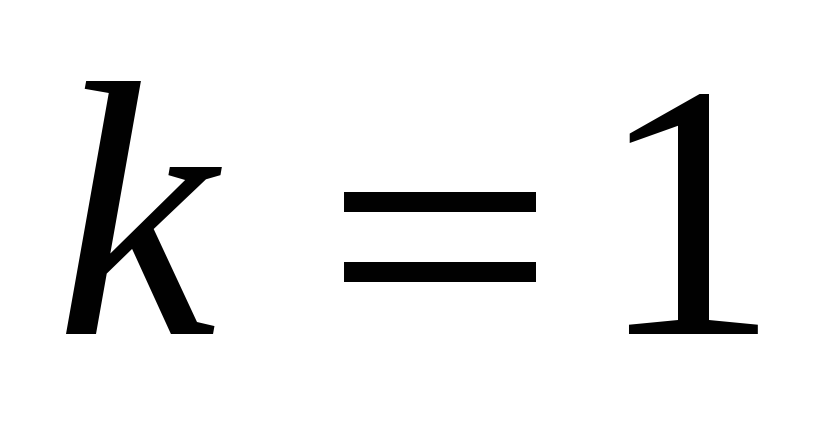,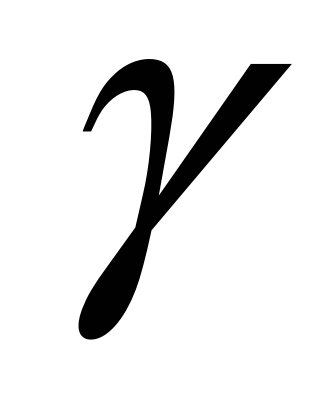=0.6,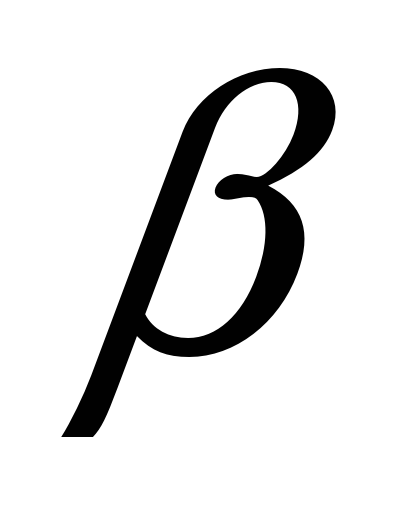= -1,= 5 and use the frequencyas a control parameter. Poincare’ sections for orbits of this system are constructed by using the excitation frequency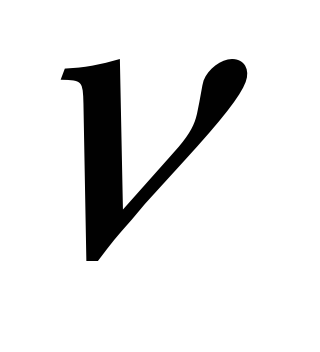. For each orbit of the system the discrete points (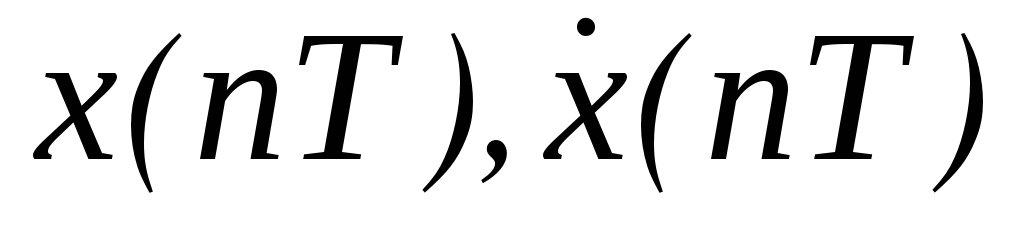) are collected at time intervals of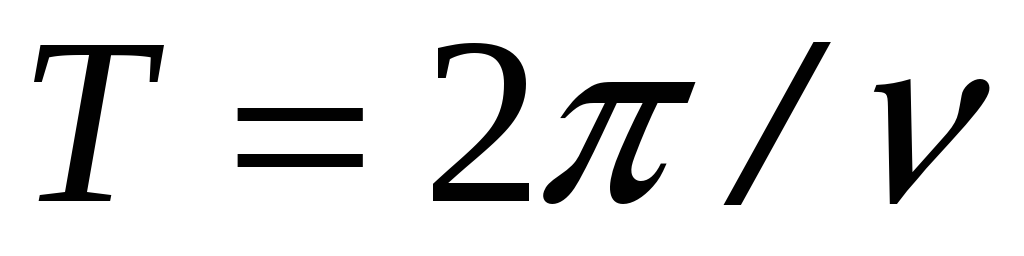(the period of the external excitation force). The bifurcation diagram shown in Figure 2 was generated by incrementing the control parameterin steps of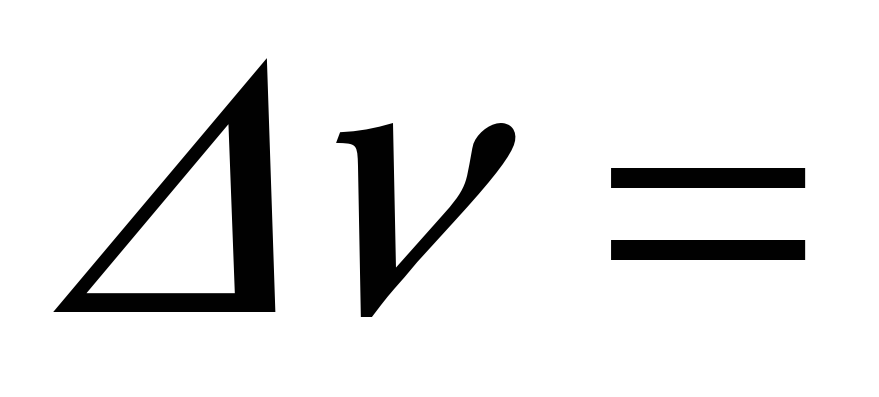0.0001. The graph consists of the points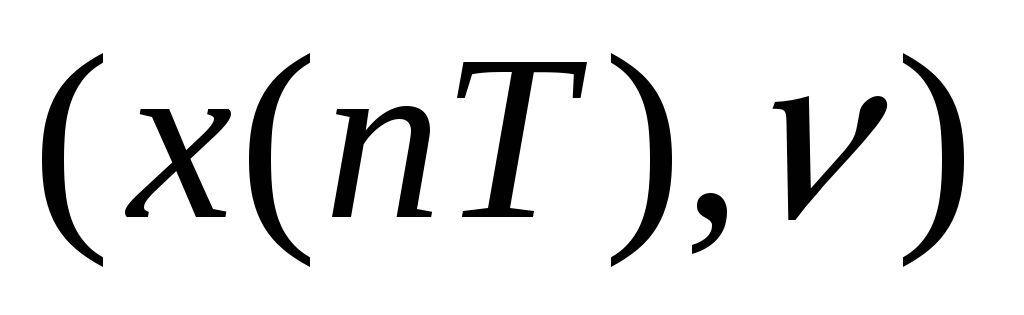, where the values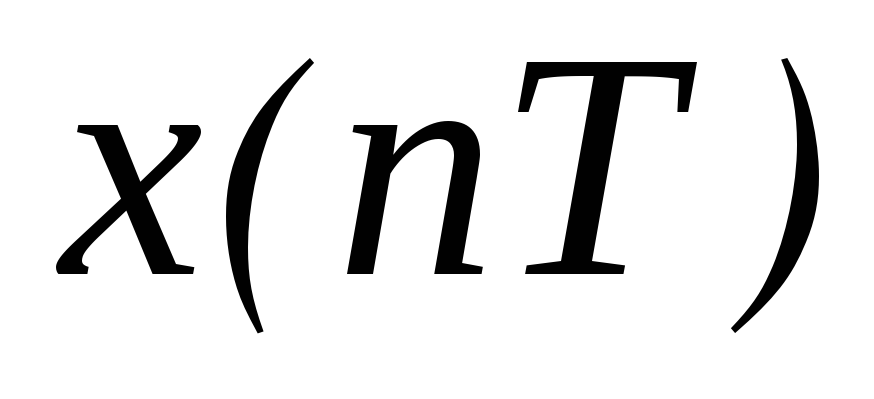correspond to the attractor realized at each value of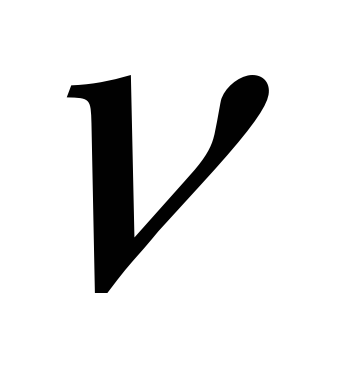.

From Figure 2 it is clear that asincreased through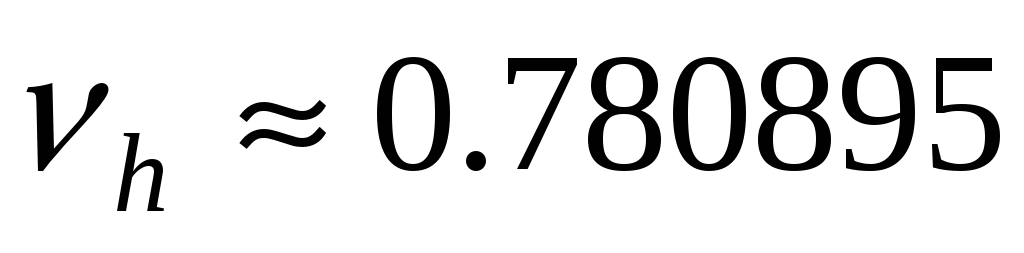, there is an abrupt transition from the point attractor to an aperiodic one, so a Hopf bifurcation of a periodic solution (the Poincare’ section consists of only one point) occurs. For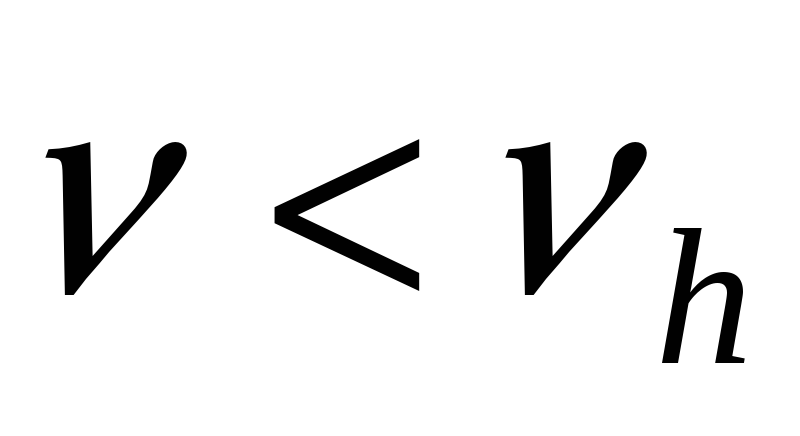, the state of the system is periodic, when the control parameter exceeds the threshold value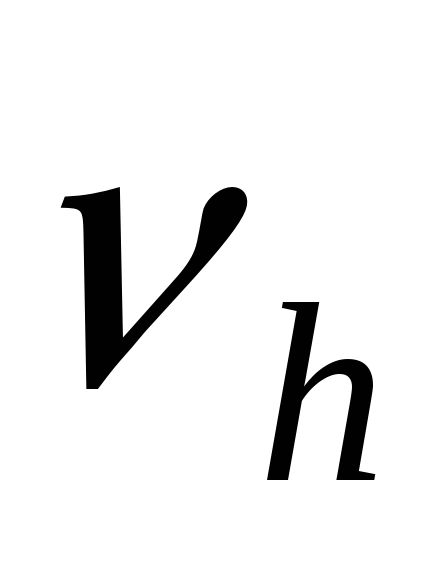, the system evolution is attracted to chaotic attractor, then the system undergoes a subcritical Hopf bifurcation. The attractors, both before and after the bifurcation, are shown in Figure 3(a, b). Figure 3(a) describes the periodic attractor with its Poincaré section consisting of one point (*) connected to=0.78. With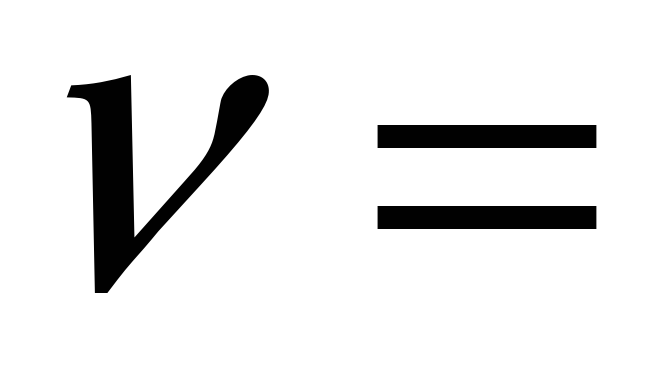0.782 a chaotic motion occurs, Figure3(b) describes its attractor. We’ll consider this case more detail below.

With the values of the frequencies, the Poincaré sections consist of one point, the motions are periodic with the period equating the one of the external force. Beyond the periodic region occupying much of the interval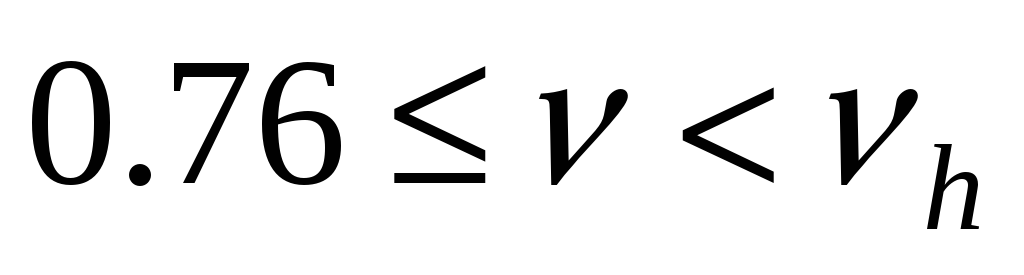, there exists a wide interval in which for certain ranges of the parameter, the displacement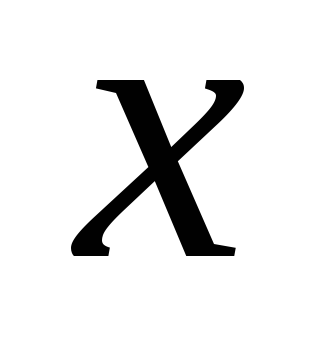takes an infinite number of values; these states are aperiodic.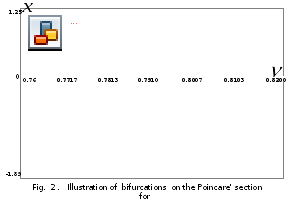It is also interesting to see that within the aperiodic regions there are narrow intervals in which the motion abruptly becomes periodic again, for example, the region around the value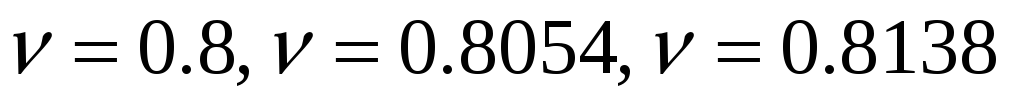....... In this interval, for every parameter,takes a finite number of values (more than one), so that the corresponding motions are subharmonic oscillations.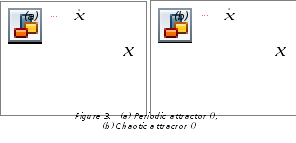Cơ sở dữ liệu được bảo vệ bởi bản quyền ©hocday.com 2019
được sử dụng cho việc quản lý

Quê hương